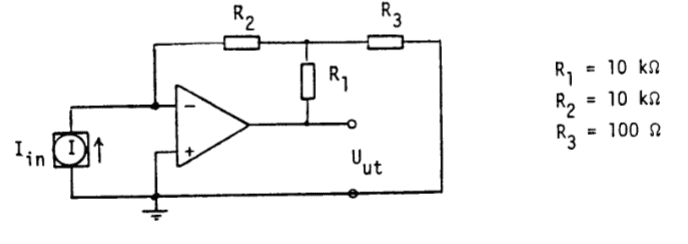# Find out voltage (OP-amp)

• DenDanne
In summary, the current flows like the image below, but calculating the output voltage for the opamp is a bit confusing because there is no current flowing through the resistor between the - input and R1.

#### DenDanne

Thread moved from the technical forums to the schoolwork forums
Summary:: Find the voltage in an OP-amp circuit with current source

I(in) = 1 uA.What I'm confused about is if there is any voltage flowing through R1. Because if there was and let's say it went downwards, then where would it go, I mean it cannot just disappear. And if it goes upwards, then it has to go to the right through R3 (right?). But then we have I(in)+I(out) = I(in) and that does not make sense that more current can just appear out of nowhere.
So my guess is that the current passes like the picture below. But then how would I calculate U(ut). I can of course calculate the voltage between R2 and R3, but the problem then is that I suppose there isn't flowing any current through R1, so I cannot really use Ohms law?
https://www.physicsforums.com/attachments/299760

DenDanne said:
What I'm confused about is if there is any voltage flowing through R1.
There's no such thing as voltage "flowing through" something. Currents flow through paths, and there are voltages between nodes in a circuit (or "across" a component).

DenDanne said:
So my guess is that the current passes like the picture below. But then how would I calculate U(ut). I can of course calculate the voltage between R2 and R3, but the problem then is that I suppose there isn't flowing any current through R1, so I cannot really use Ohms law?
In a circuit with an ideal opamp, it's important to remember the fundamentals. An ideal opamp IC has very high input impedance at its +/- inputs, and the negative feedback causes the voltage difference between those +/- inputs to be zero.

So in this circuit, the - input terminal is at the same ground potential as the + input, right? And since the input impedance of the - input is high, all of that source current is going to flow through which resistor first? And doing that causes the voltage between R1, R2 and R3 to be what? And how do you keep going then to finish calculating the output voltage for the opamp?

See if you can use those hints to get farther in this problem...Last edited:
•DaveE